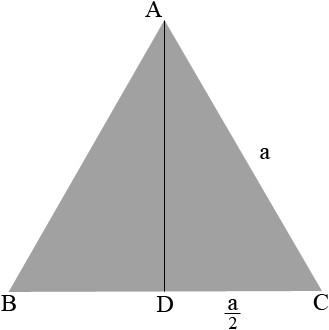SEARCH HOMEMath Central Quandaries & QueriesQuestion from saheed, a student: how many tonnes of gravel and sand,bags of cements will be required to concrete 5feet depth/deep of 4feet equilateral triangle?Hi,

Suppose you have an equilateral triangle $ABC$ with sides of length $a$ feet. Let $D$ be the midpoint of $BC$ and join $a$ to $D.$$ADC$ is a right triangle so you can use Pythagoras theorem to find the length of $AD,$ which is the height of the triangle $ABC.$ Then the area of the triangle $ABC$ is $\large \frac{a}{2} \times \normalsize |AD|$ square feet.

In your case $a = 4$ feet. Find the area of the triangle in square feet and multiply by the depth, 5 feet to obtain the volume in cubic feet. Contractors are accustomed to working in cubic yards so divide the volume in cubic feet by 27 to obtain the volume in cubic yards.

The amount of cement and aggregate you need will depend on the type of aggregate and cement as well as the ratio of cement to aggregate you plan to use. You need to talk to someone where you plan to buy your supplies.

HarleyMath Central is supported by the University of Regina and The Pacific Institute for the Mathematical Sciences.